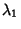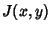## Lyapunov Characteristic Number

Given a Lyapunov Characteristic Exponent, the corresponding Lyapunov characteristic numberis defined as(1)

For an-dimensional linear Map,(2)

The Lyapunov characteristic numbers, ...,are the Eigenvalues of the Map Matrix. For an arbitrary Map(3)(4)

the Lyapunov numbers are the Eigenvalues of the limit(5)

whereis the Jacobian(6)

Iffor all, the system is not Chaotic. Ifand the Map is Area-Preserving (Hamiltonian), the product of Eigenvalues is 1.